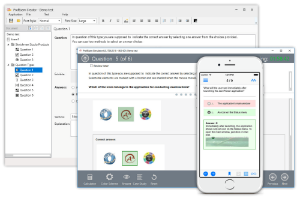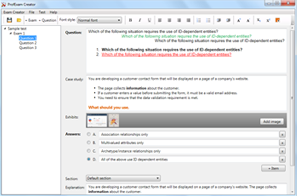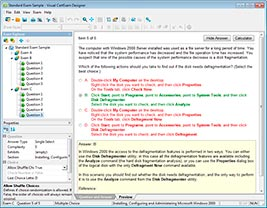# Download Java SE 8 Programmer II.passit4sure.1z0-809.2019-02-04.1e.54q.vcex

## File Info

 Exam Java SE 8 Programmer II Number 1z0-809 File Name Java SE 8 Programmer II.passit4sure.1z0-809.2019-02-04.1e.54q.vcex Size 1.61 Mb Posted February 04, 2019 Downloads 24

### How to open VCEX & EXAM Files?

Files with VCEX & EXAM extensions can be opened by ProfExam Simulator.

Discount: 20%

## Demo Questions

Question 1
Given:
IntStream stream = IntStream.of (1,2,3);
IntFunction<Integer> inFu= x -> y -> x*y;            //line n1
IntStream newStream = stream.map(inFu.apply(10));    //line n2
newStream.forEach(System.output::print);
Which modification enables the code fragment to compile?

• A: Replace line n1 with:
IntFunction<UnaryOperator> inFu = x -> y -> x*y;
• B: Replace line n1 with:
IntFunction<IntUnaryOperator> inFu = x -> y -> x*y;
• C: Replace line n1 with:
BiFunction<IntUnaryOperator> inFu = x -> y -> x*y;
• D: Replace line n2 with:
IntStream newStream = stream.map(inFu.applyAsInt (10));

Question 2
Given the code fragment:
public class Foo {
public static void main (String [ ] args) {
Map<Integer, String> unsortMap = new HashMap< > ( );
unsortMap.put (10, “z”);
unsortMap.put (5, “b”);
unsortMap.put (1, “d”);
unsortMap.put (7, “e”);
unsortMap.put (50, “j”);
Map<Integer, String> treeMap = new TreeMap <Integer, String> (new
Comparator<Integer> ( )  {
@Override public int compare (Integer o1, Integer o2) {return o2.compareTo
(o1); }  } );
treeMap.putAll (unsortMap);
for (Map.Entry<Integer, String> entry : treeMap.entrySet () ) {
System.out.print (entry.getValue () + “ “);
}
}
}
What is the result?

• A: A compilation error occurs.
• B: d b e z j
• C: j z e b d
• D: z b d e j

Question 3
Given:
class Bird  {
public void fly ()  {  System.out.print(“Can fly”); }
}
class Penguin extends Bird  {
public void fly ()  {  System.out.print(“Cannot fly”); }
}
and the code fragment:
class Birdie {
public static void main (String [ ] args)  {
fly( ( ) -> new Bird ( ));
fly (Penguin : : new);
}
/* line n1 */
}
Which code fragment, when inserted at line n1, enables the Birdie class to compile?

• A: static void fly (Consumer<Bird> bird)  {
bird :: fly ();
}
• B: static void fly (Consumer<? extends Bird> bird)  {
bird.accept( ) fly ();
}
• C: static void fly (Supplier<Bird> bird)  {
bird.get( ) fly ();
}
• D: static void fly (Supplier<? extends Bird> bird)  {
LOST

Question 4
Given:
1. abstract class Shape  {
2.     Shape ( )   { System.out.println  (“Shape”);     }
3.     protected void area ( ) { System.out.println  (“Shape”);     }
4. }
5.
6. class Square extends Shape  {
7.     int side;
8.     Square int side {
9.        /* insert code here */
10.         this.side = side;
11.     }
12.     public void area ( ) { System.out.println  (“Square”);     }
13.     }
14. class Rectangle extends Square {
15.     int len, br;
16.     Rectangle (int x, int y)   {
17.         /* insert code here */
18.         len = x, br = y;
19.     }
20. void area ( ) { System.out.println  (“Rectangle”);     }
21. }
Which two modifications enable the code to compile?

• A: At line 1, remove abstract
• B: At line 9, insert super ( );
• C: At line 12, remove public
• D: At line 17, insert super (x);
• E: At line 17, insert super (); super.side = x;
• F: At line 20, use public void area ( ) {

Question 5
Given:
class Sum extends RecursiveAction   {                 //line n1
static final int THRESHOLD_SIZE  = 3;
int stIndex, lstIndex;
int [ ] data;
public Sum (int [ ]data, int start, int end)   {
this.data = data;
this stIndex = start;
this. lstIndex = end;
}
protected void compute ( )    {
int sum = 0;
if (lstIndex – stIndex <= THRESHOLD_SIZE)  {
for (int i = stIndex; i < lstIndex; i++)   {
sum += data [i];
}
System.out.println(sum);
} else {
new Sum (data, stIndex + THRESHOLD_SIZE, lstIndex).fork( );
new Sum (data, stIndex,
Math.min  (lstIndex, stIndex + THRESHOLD_SIZE)
).compute ();
}
}
}
and the code fragment:
ForkJoinPool fjPool = new ForkJoinPool ( );
int data [ ] = {1, 2, 3, 4, 5, 6, 7, 8, 9, 10}
fjPool.invoke (new Sum (data, 0, data.length));
and given that the sum of all integers from 1 to 10 is 55.
Which statement is true?

• A: The program prints several values that total 55.
• B: The program prints 55.
• C: A compilation error occurs at line n1.
• D: The program prints several values whose sum exceeds 55.

Question 6
Given the definition of the Country class:
public class country {
public enum Continent {ASIA, EUROPE}
String name;
Continent region;
public Country (String na, Continent reg)   {
name = na, region = reg;
}
public String getName () {return name;}
public Continent getRegion () {return region;}
}
and the code fragment:
List<Country> couList = Arrays.asList (
new Country (“Japan”, Country.Continent.ASIA),
new Country (“Italy”, Country.Continent.EUROPE),
new Country (“Germany”, Country.Continent.EUROPE));
Map<Country.Continent, List<String>> regionNames = couList.stream ()
.collect(Collectors.groupingBy (Country ::getRegion,
Collectors.mapping(Country::getName, Collectors.toList()))));
System.out.println(regionNames);

• A: {EUROPE = [Italy, Germany], ASIA = [Japan]}
• B: {ASIA = [Japan], EUROPE = [Italy, Germany]}
• C: {EUROPE = [Germany, Italy], ASIA = [Japan]}
• D: {EUROPE = [Germany], EUROPE = [Italy], ASIA = [Japan]}

Question 7
Given the code fragment:
Map<Integer, String> books = new TreeMap<>();
books.put (1007, “A”);
books.put (1002, “C”);
books.put (1001, “B”);
books.put (1003, “B”);
System.out.println (books);
What is the result?

• A: {1007 = A, 1002 = C, 1001 = B, 1003 = B}
• B: {1001 = B, 1002 = C, 1003 = B, 1007 = A}
• C: {1002 = C, 1003 = B, 1007 = A}
• D: {1007 = A, 1001 = B, 1003 = B, 1002 = C}

Question 8
Given the content of /resourses/Message.properties:
welcome1=”Good day!”
and given the code fragment:
Properties prop = new Properties ();
FileInputStream fis = new FileInputStream (“/resources/Message.properties”);
prop.load(fis);
System.out.println(prop.getProperty(“welcome1”));
System.out.println(prop.getProperty(“welcome2”, “Test”));//line n1
System.out.println(prop.getProperty(“welcome3”));
What is the result?

• A: Good day!
Test
followed by an Exception stack trace
• B: Good day!
followed by an Exception stack trace
• C: Good day!
Test
null
• D: A compilation error occurs at line n1.

Question 9
Which action can be used to load a database driver by using JDBC3.0?

• A: Add the driver class to the META-INF/services folder of the JAR file.
• B: Include the JDBC driver class in a jdbc.properties file.
• C: Use the java.lang.Class.forName method to load the driver class.
• D: Use the DriverManager.getDriver method to load the driver class.

Question 10
Given the code fragment:
Path p1 = Paths.get(“/Pics/MyPic.jpeg”);
System.out.println (p1.getNameCount() +
“:” + p1.getName(1) +
“:” + p1.getFileName());
Assume that the Pics directory does NOT exist.
What is the result?

• A: An exception is thrown at run time.
• B: 2:MyPic.jpeg: MyPic.jpeg
• C: 1:Pics:/Pics/ MyPic.jpeg
• D: 2:Pics: MyPic.jpeg

CONNECT US##### PROFEXAM WITH A 20% DISCOUNT

You can buy ProfExam with a 20% discount..

HOW TO OPEN VCEX AND EXAM FILES

Use ProfExam Simulator to open VCEX and EXAM filesHOW TO OPEN VCE FILES

Use VCE Exam Simulator to open VCE files